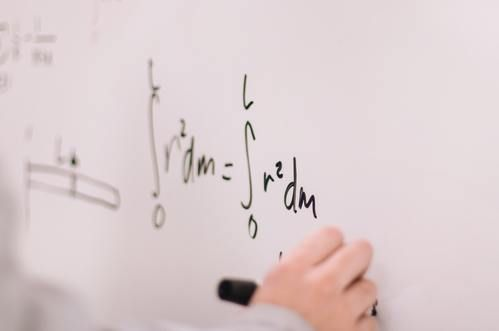# Homeschool Classes

These classes are intended for homeschool families who want to outsource their children for math classes. These classes are hosted at Simple Faith Calvary Chapel and are faith based.

## Summer Courses 2022

### High School Math Prep

Target Student: This summer course is intended to prepare students who are close to or are beginning highschool math classes. Your student should enroll in this summer course if they plan to enroll in either Math 76 or Pre-Algebra in the fall.

Course Description: This summer course will cover a new topic each week. Over the course of one hour each week, students will be introduced to a concept with the intent to strengthen their skill in that concept in preparation for the upcoming school year. The first part of class will be an introduction to the topic and how it applies to real life situations. The latter portion of class will consist of practical practice with the concept through fun, interactive activities.

Tentative Schedule of Topics:
July 8th: Divisibility Rules
July 15th: Least Common Multiple
July 22th: Fractions (Adding and Subtracting) July 29th: Fractions, percents, decimals August 5th: Perimeter
August 12th: Area

### High School Math Refresher

Target Student: This summer course is intended to prepare students who have already taken some high school level math classes. Your student should enroll in this summer course if they plan to enroll in either Algebra 1 or Algebra 2 in the fall.

Course Description: This summer course will cover a new topic each week. Over the course of one hour each week, students will be introduced to a concept with the intent to strengthen their skill in that concept in preparation for the upcoming school year. The first part of class will be an introduction to the topic and how it applies to real life situations. The latter portion of class will consist of practical practice with the concept through fun, interactive activities.

Tentative Schedule of Topics:
July 8th: Fractions (Adding, subtracting, multiplying, and dividing) July 15th: Circumference and Area of Circles
July 22th: Order of Operations
July 29th: Solving Equations with one unknown
August 5th: Graphing Linear Equations part 1 August 12th: Graphing Linear Equations part 2

## Fall Courses 2022

### Math 7/6

Curriculum: Saxon Math 76 Third Edition
ISBN:1-56577-153-2

Course Description: Math 76 is appropriate for any age student preparing to enter Pre-Algebra the following year. Students are introduced to problems that model simple real life situations. Math 76 covers concepts such as least common multiple, greatest common factor, fractions, mixed numbers, decimals, percents, common denominators, order of operations, area, and perimeter. This class helps students practice the fundamentals principles that algebra is built upon.

### Pre-Algebra

Curriculum: Saxon Pre-Algebra Third Edition
ISBN: 1-56577-149-4

Course Description: Pre-Algebra introduces students to the foundation of algebra. Throughout this course students will begin to strengthen their algebraic problem solving skills. Students will be introduced to more realistic real life problems. Some of the concepts covered in this course include solving equations with one unknown, solving equations symbolically, simplifying variable expressions with exponents, operations with negative numbers, graphing linear equations, percent and fraction word problems, surface area, and volume.

### Algebra 1

Curriculum: Saxon Algebra 1 Third Edition
ISBN:1-56577-134-6

Course Description: Algebra 1 continues to develop students' algebraic skills. Algebra 1 builds upon what students have learned and practiced in Pre-Algebra. Algebra 1 introduces students to concepts such as solving multivariable equations, solving multiple equations using substitution or elimination, simplifying expressions with positive and negative exponents, distance word problems, multiplication of polynomials, and probability.

### Algebra 2

Curriculum: Saxon Algebra 2 Third Edition
ISBN: 1-56577-140-0

Course Description: Algebra 2 covers more complex algebraic concepts that help students more closely model real life situations. This course will prepare students for college level math courses. Concepts that will be covers in Algebra 2 include the Pythagorean Theorem, ratio word problems, value word problems, monomial and trinomial factoring, chemical compound word problems, chemical mixture problems, the sine function, cosine function, tangent function, polar coordinates, rectangular coordinates, vectors, non-linear equations, graphs of nonlinear equation, logarithms and antilogarithms.

## Cost

(All suggested donation amounts)
Cost for these courses is as follows:
\$60 per month for the first child that is enrolled
\$50 per month per child thereafter
(Pertains to immediate family unit only; does not pertain to friends or extended family members.)